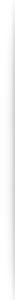## Quick Way to Calculate Stamp Duty Payable for Buying Singapore Property

Stamp Duty payable by Buyer of Singapore Property

- First \$180,000 is 1% .
- Second \$180,000 is 2%.
- Thereafter, 3% of the remaining property price.

For property price more than \$360K, a quick way to compute the stamp duty is to consider a flat rate of 3% on the total property price, then subtract the excess amount for the first \$180K and next \$180K which is \$5400.

Quick Formula:
Stamp Duty = 3% x Property price - \$5400

Example 1: Property Price = \$1M
Stamp Duty = 3% of \$1M - \$5400 = \$30,000 - \$5400 = \$24,600

Example 2: Property Prcie = \$600K
Stamp Duty = 3% of \$600K - \$5400 = \$12,600

For property price less than \$360K, it's 1% for first \$180K and 2% for the next \$180K.

Example 3: Property Price = \$280K

Stamp Duty = 1% x \$180,000 + 2% x \$100,000 = \$3800

Example 4: Property Price = \$160K

Stamp Duty = 1% x \$160,000 = \$1600

(The discounts given to the first 360K is to take care of people from the lower income group who are buying a small property like a 3-rm HDB flat.)Kenneth Chan 曾健德
Associate Division Director
ERA REALTY NETWORK PTE LTD
CEA Licence No.:
L3002382K / R024796D
+(65) 9100 6270